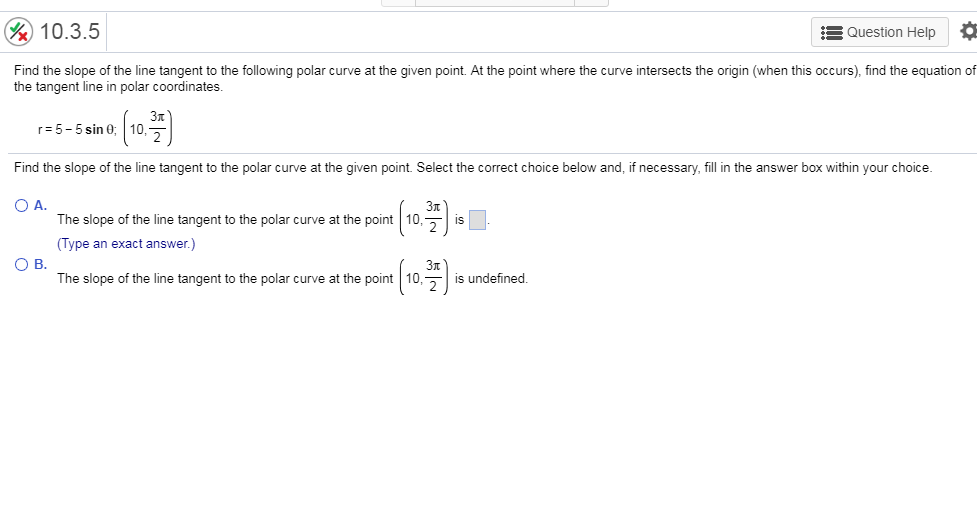# &10.3.5 Question Help Find the slope of the line tangent to the following polar curve at the given point. At the point where the curve intersects the origin (when this occurs), find the equation of the tangent line in polar coordinates. 5-5sin 10 Find the slope of the line tangent to the polar curve at the given point. wer box within your cho 0 A. The slope of the line tangent to the polar curve at the point 10,is Type an exact answer.) gettothe polar curve at the port (103m)- O B. 3π The slope of the line tangent to the polar curve at the point | 10,지 is undefined

Question

10.3.5help_outlineImage Transcriptionclose&10.3.5 Question Help Find the slope of the line tangent to the following polar curve at the given point. At the point where the curve intersects the origin (when this occurs), find the equation of the tangent line in polar coordinates. 5-5sin 10 Find the slope of the line tangent to the polar curve at the given point. wer box within your cho 0 A. The slope of the line tangent to the polar curve at the point 10,is Type an exact answer.) gettothe polar curve at the port (103m)- O B. 3π The slope of the line tangent to the polar curve at the point | 10,지 is undefined fullscreen

### Want to see the step-by-step answer?